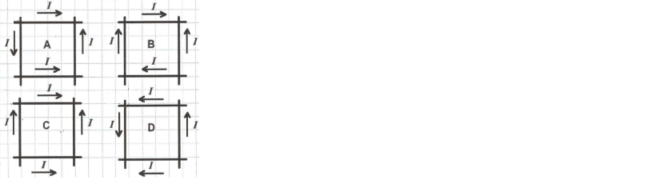# Problem: The figure shows four different sets of insulated wires that cross each other at right angles without making electrical contact. The magnitude of the current is the same in all the wires, and the direction of current flow are as indicated. For which (if any) configuration will the magnetic field at the center of the square formed by the wires be equal to zero? A) A B) B C) C D) D E) The field is not equal to zero in any of these cases.

###### FREE Expert Solution
96% (493 ratings)
###### Problem Details

The figure shows four different sets of insulated wires that cross each other at right angles without making electrical contact. The magnitude of the current is the same in all the wires, and the direction of current flow are as indicated. For which (if any) configuration will the magnetic field at the center of the square formed by the wires be equal to zero?

A) A

B) B

C) C

D) D

E) The field is not equal to zero in any of these cases.